Test: Production and Costs- Match Based Type Questions

# Test: Production and Costs- Match Based Type Questions

Test Description

## 15 Questions MCQ Test Economics Class 11 | Test: Production and Costs- Match Based Type Questions

Test: Production and Costs- Match Based Type Questions for Commerce 2023 is part of Economics Class 11 preparation. The Test: Production and Costs- Match Based Type Questions questions and answers have been prepared according to the Commerce exam syllabus.The Test: Production and Costs- Match Based Type Questions MCQs are made for Commerce 2023 Exam. Find important definitions, questions, notes, meanings, examples, exercises, MCQs and online tests for Test: Production and Costs- Match Based Type Questions below.
Solutions of Test: Production and Costs- Match Based Type Questions questions in English are available as part of our Economics Class 11 for Commerce & Test: Production and Costs- Match Based Type Questions solutions in Hindi for Economics Class 11 course. Download more important topics, notes, lectures and mock test series for Commerce Exam by signing up for free. Attempt Test: Production and Costs- Match Based Type Questions | 15 questions in 30 minutes | Mock test for Commerce preparation | Free important questions MCQ to study Economics Class 11 for Commerce Exam | Download free PDF with solutions
 1 Crore+ students have signed up on EduRev. Have you?
Test: Production and Costs- Match Based Type Questions - Question 1

### Identify the correct pair of items from the following Columns I and II: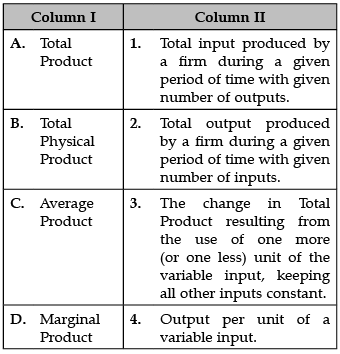Detailed Solution for Test: Production and Costs- Match Based Type Questions - Question 1

A. Total Product: Total product (definition) the total quantity of output produced by firm. marginal product (definition) is the extra or additional output resulting from one additional unit of the variable input (usually labor).

B. Total Physical Product: The total quantity of output produced by a firm for a given quantity of inputs. Total physical product is the total production of output by a firm based on the quantity of inputs used.

C. Average Product: It is defined as the output per unit of factor inputs or the average of the total product per unit of input and can be calculated by dividing the Total Product by the inputs (variable factors). Average Product = Total Product/ Units of Variable Factor Input.

D. Marginal Product: Marginal productivity or marginal product refers to the extra output, return, or profit yielded per unit by advantages from production inputs. Inputs can include things like labor and raw materials. This means that the cost advantage usually diminishes for each additional unit of output produced.

Test: Production and Costs- Match Based Type Questions - Question 2

### Identify the correct pair of terms and definitions from the following Columns I and II: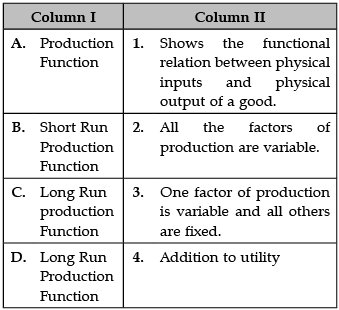Detailed Solution for Test: Production and Costs- Match Based Type Questions - Question 2

A. Production Function: Production function, in economics, equation that expresses the relationship between the quantities of productive factors (such as labour and capital) used and the amount of product obtained.

B. Short Run Production Function: The short-run production function defines the relationship between one variable factor (keeping all other factors fixed) and the output.

C. Long Run Production Function: Long run production function refers to that time period in which all the inputs of the firm are variable. It can operate at various activity levels because the firm can change and adjust all the factors of production and level of output produced according to the business environment.

D. Long Run Production Function: Long run production function refers to that time period in which all the inputs of the firm are variable. It can operate at various activity levels because the firm can change and adjust all the factors of production and level of output produced according to the business environment.

Test: Production and Costs- Match Based Type Questions - Question 3

### Identify the correct pair of from the following Columns I and II: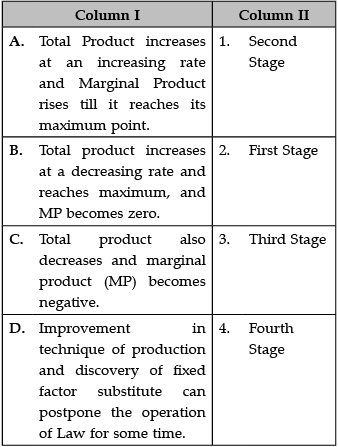Detailed Solution for Test: Production and Costs- Match Based Type Questions - Question 3

A. Second Stage: The second stage of Rostow's Stages of Development is generally described as “prerequisites for rapid economic growth.” It is the intermediary step between a traditional, agrarian society and industrial explosion. It primarily involves external investment and a much greater exploitation of natural resources.

B. First Stage: The first stage in the business cycle is expansion. In this stage, there is an increase in positive economic indicators such as employment, income, output, wages, profits, demand, and supply of goods and services.

C. Third Stages: Total production also decreases and marginal product (MP) becomes negative.

D. Fourth Stage: The four phases of the business cycle are peak, recession, trough, and expansion.

Test: Production and Costs- Match Based Type Questions - Question 4

Identify the correct pair of items from the following Columns I and II: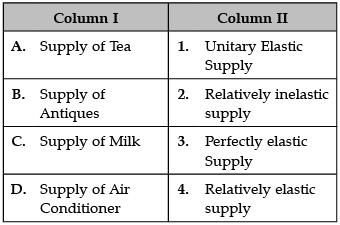Detailed Solution for Test: Production and Costs- Match Based Type Questions - Question 4

A. Supply of Tea: Empirical results show that demand for green tea, black tea, and tea beverage are own-price elastic while coffee and coffee beverage are own-price inelastic.

B. Supply of Antiques: The supply of antiques is highly inelastic.Thus,increases in demand would have a small effect on price. A positive cross-elasticity of demand between two goods indicates that the two goods are both normal goods.

C. Supply of Milk: Basic necessities are generally said to be price inelastic in comparison with luxury goods. In particular, fluid milk has long been regarded as one of the most price inelastic commodities in many countries. According to their findings, the value for the milk category is 0.59, which is relatively inelastic.

D. Supply of Air Conditioner: The supply for air conditioners is perfectly inelastic and it is shown by a vertical curve. A perfectly inelastic supply means that the quantity of conditioners is limited as the supplies does not have any inventory reserves.

Test: Production and Costs- Match Based Type Questions - Question 5

Identify the correct pair of items from the following Columns I and II: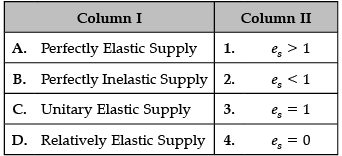Detailed Solution for Test: Production and Costs- Match Based Type Questions - Question 5

A. Perfectly Elastic Supply: PES = infinity: Supply is perfectly elastic. An decrease in prices will lead to zero units produced.

B. Perfectly Inelastic Supply: Perfectly inelastic supply is when the PES formula equals 0. That is, there is no change in quantity supplied when the price changes. Examples include products that have limited quantities, such as land or painting from deceased artists.

C. Unitary Elastic Supply: Unitary elasticities indicate proportional responsiveness of demand. In other words, the percent change in quantity demanded is equal to the percent change in price, so the elasticity equals 1.

D. Relatively Elastic Supply: A price elasticity supply greater than 1 means supply is relatively elastic, where the quantity supplied changes by a larger percentage than the price change. An example would be a product that's easy to make and distribute, such as a fidget spinner.

Test: Production and Costs- Match Based Type Questions - Question 6

Identify the correct pair of items from the following Columns I and II: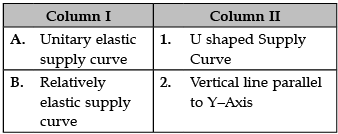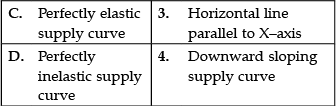Detailed Solution for Test: Production and Costs- Match Based Type Questions - Question 6

A. Unitary elastic supply Curve: Rectangular hyperbola is a curve under which all rectangular areas are equal. When the elasticity of demand is equal to unity (ed = 1) at all points of demand curve, then the demand curve is rectangular hyperbola.

B. Relatively elastic supply curve: PES = 0: The supply curve is vertical; there is no response of demand to prices. Supply is “perfectly inelastic.” PES = ∞ (i.e., infinity): The supply curve is horizontal; there is extreme change in demand in response to very small change in prices. Supply is “perfectly elastic.”

C. Perfectly elastic supply curve: The horizontal lines show that an infinite quantity will be demanded or supplied at a specific price. This illustrates the cases of a perfectly (or infinitely) elastic demand curve and supply curve.

D. Perfectly inelastic supply curve: The shape of a perfectly inelastic supply curve is shown below: The supply curve is vertical at the specific quantity supplied of Qs. This curve highlights that any change in price does not cause a change in the quantity supplied.

Test: Production and Costs- Match Based Type Questions - Question 7

Identify the correct pair of items from the following Columns I and II: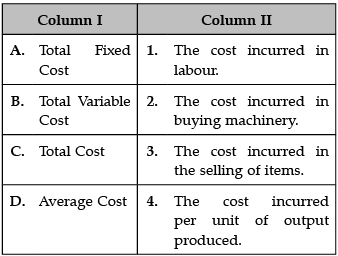Detailed Solution for Test: Production and Costs- Match Based Type Questions - Question 7

A. Total Fixed Cost: Total fixed cost (TFC) is that cost which does not change with change in the level of output. Eg: Depreciation, Rent, Salaries, Insurance etc.

B. Total Variable Cost: Total variable cost (TVC) is that cost which changes as the level of output changes. Eg: Piece Labour Rate, Freight charges Outward, Raw Material Cost, Electricity etc.

C. Total Cost: Total cost refers to the total expense incurred in reaching a particular level of output; if such total cost is divided by the quantity produced, average or unit cost is obtained.

D. Average Cost: The average cost is the total cost divided by the number of goods produced. It is also equal to the sum of average variable costs and average fixed costs.

Test: Production and Costs- Match Based Type Questions - Question 8

Identify the correct pair of items from the following Columns I and II: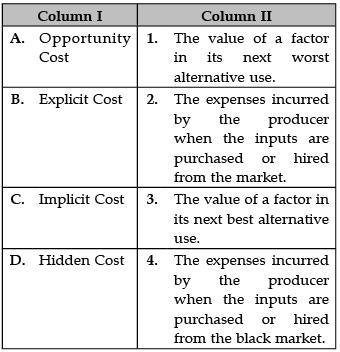Detailed Solution for Test: Production and Costs- Match Based Type Questions - Question 8

A. Opportunity Cost: Opportunity costs represent the potential benefits an individual, investor, or business misses out on when choosing one alternative over another.

B. Explicit Cost: Explicit costs are normal business costs that appear in the general ledger and directly affect a company's profitability.

C. Implicit Cost: An implicit cost is any cost that has already occurred but not necessarily shown or reported as a separate expense.

D. Hidden Cost: A hidden cost is a cost imposed by a transaction or activity that is not immediately apparent simply by looking at the trade occurring.

Test: Production and Costs- Match Based Type Questions - Question 9

Identify the correct pair of items from the following Columns I and II: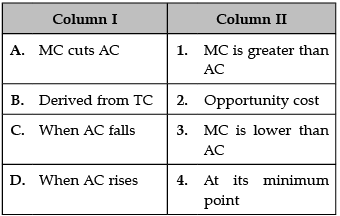Detailed Solution for Test: Production and Costs- Match Based Type Questions - Question 9

A. MC cuts AC: MC curve cuts AC curve at its lowest point.

B. Derived from TC: Total cost (TC) is the minimum dollar cost of producing some quantity of output. Thus, the total fixed cost equals Kr.

C. When AC falls: AC can fall, when MC is rising. However, it is possible only when MC is less than AC. It means that as long as MC curve is below the AC curve, AC will fall even if MC is rising.

D. When AC rises: When AC rises with increase in output, MC is higher than AC, i.e., MC curve lies above the AC curve.

Test: Production and Costs- Match Based Type Questions - Question 10

Identify the correct pair of items from the following Columns I and II: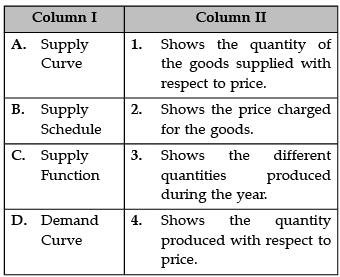Detailed Solution for Test: Production and Costs- Match Based Type Questions - Question 10

A. Supply Curve: The supply curve is a graphic representation of the correlation between the cost of a good or service and the quantity supplied for a given period.

B. Supply Schedule: A supply schedule is a table that shows the quantity supplied at each price. A supply curve is a graph that shows the quantity supplied at each price. Sometimes the supply curve is called a supply schedule because it is a graphical representation of the supply schedule.

C. Supply Function: The supply function is the mathematical expression of the relationship between supply and those factors that affect the willingness and ability of a supplier to offer goods for sale.

D. Demand Curve: The demand curve is a graphical representation of the relationship between the price of a good or service and the quantity demanded for a given period of time.

Test: Production and Costs- Match Based Type Questions - Question 11

Identify the correct pair of items from the following Columns I and II: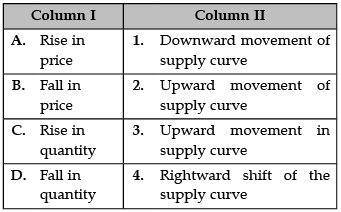Detailed Solution for Test: Production and Costs- Match Based Type Questions - Question 11

A. Rise in price: The supply curve will move upward from left to right, which expresses the law of supply: As the price of a given commodity increases, the quantity supplied increases (all else being equal). In other words, supply will increase. Technology is a leading cause of supply curve shifts.

B. Fall in price: If there is an decrease in supply ( S) the supply curve moves to the LEFT. At the same prices, the quantities supplied will be smaller.

C. Rise in quantity: The supply curve will move upward from left to right, which expresses the law of supply: As the price of a given commodity increases, the quantity supplied increases (all else being equal). In other words, supply will increase. Technology is a leading cause of supply curve shifts.

D. Fall in quantity: The downward shift represents the fact that supply often increases when the costs of production decrease, so producers don't need to get as high of a price as before in order to supply a given quantity of output.

Test: Production and Costs- Match Based Type Questions - Question 12

Identify the correct pair of items from the following Columns I and II: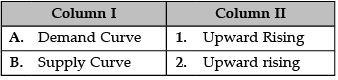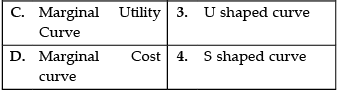Detailed Solution for Test: Production and Costs- Match Based Type Questions - Question 12

A. Demand Curve: The demand curve is a graphical representation of the relationship between the price of a good or service and the quantity demanded for a given period of time.

B. Supply Curve: The supply curve is a graphic representation of the correlation between the cost of a good or service and the quantity supplied for a given period.

C. Marginal Utility Curve: Marginal utility is the added satisfaction that a consumer gets from having one more unit of a good or service.

D. Marginal Cost Curve: A curve that graphically represents the relation between the marginal cost incurred by a firm in the short-run product of a good or service and the quantity of output produced.

Test: Production and Costs- Match Based Type Questions - Question 13

Identify the correctly matched items from Column I to that of Column II: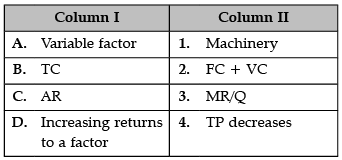Detailed Solution for Test: Production and Costs- Match Based Type Questions - Question 13

A. Variable factor: Variable factors are those that do change with output, which means more are employed when production increases, and less when production decreases. Typical variable factors include labour, energy, and raw materials directly used in production.

B. TC: The total cost formula is used to combine the variable and fixed costs of providing goods to determine a total. The formula is: Total cost = (Average fixed cost x average variable cost) x Number of units produced.

C. AR: Accounts Receivable Equation for Turnover = Net Sales on Credit / Average Accounts Receivable.

D. Increasing returns to a factor: The law of Increasing Returns is also known as the Law of Diminishing Costs. According to this law when more and more units of variable factors are employed while other factors are kept constant, there will be an increase of production at a higher rate.

Test: Production and Costs- Match Based Type Questions - Question 14

Identify the correctly matched items from Column I to that of Column II: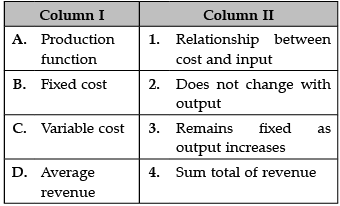Detailed Solution for Test: Production and Costs- Match Based Type Questions - Question 14

A. Production function: Production function, in economics, equation that expresses the relationship between the quantities of productive factors (such as labour and capital) used and the amount of product obtained.

B. Fixed Cost: A fixed cost is a cost that does not change with an increase or decrease in the amount of goods or services produced or sold. Fixed costs are expenses that have to be paid by a company, independent of any specific business activities.

C. Variable cost: A variable cost is a corporate expense that changes in proportion to how much a company produces or sells. Variable costs increase or decrease depending on a company's production or sales volume, they rise as production increases and fall as production decreases. A variable cost can be contrasted with a fixed cost.

D. Average revenue: Average revenue is the mathematical average of revenue earned per unit or per user. The average revenue per unit or user (ARPU) allows a company's investors or management team to analyze revenue generation capability and forecast future growth.

Test: Production and Costs- Match Based Type Questions - Question 15

Identify the correctly matched statements from Column I to that of Column II: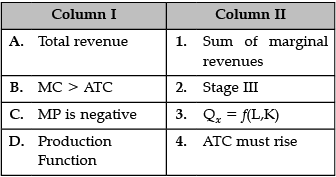Detailed Solution for Test: Production and Costs- Match Based Type Questions - Question 15

A. Total revenue: Total revenue is the full amount of total sales of goods and services. It is calculated by multiplying the total amount of goods and services sold by the price of the goods and services.

B. MC > ATC: The relationship between the ATC and MC. Whenever MC is less than ATC, ATC is falling. Whenever MC is greater than ATC, ATC is rising. When ATC reaches its minimum point, MC = ATC.

C. MP is negative: MP is that rate. At the point where TP is at its maximum, MP = 0, the point at which it crosses the x- axis. After this point, MP is actually negative, meaning that TP is falling.

D. Production Function: Production function, in economics, equation that expresses the relationship between the quantities of productive factors (such as labour and capital) used and the amount of product obtained.

## Economics Class 11

201 videos|199 docs|64 tests
 Use Code STAYHOME200 and get INR 200 additional OFF Use Coupon Code
Information about Test: Production and Costs- Match Based Type Questions Page
In this test you can find the Exam questions for Test: Production and Costs- Match Based Type Questions solved & explained in the simplest way possible. Besides giving Questions and answers for Test: Production and Costs- Match Based Type Questions, EduRev gives you an ample number of Online tests for practice

## Economics Class 11

201 videos|199 docs|64 tests